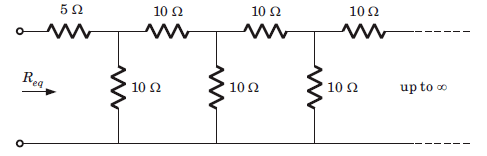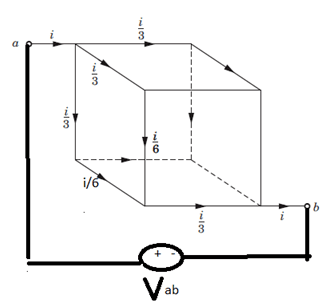## Semiconductor Devices Questions and Answers Part-1

1. A solid copper sphere, 10 cm in diameter is deprived of 1020 electrons by a charging scheme. The charge on the sphere is _________
a) 160.2 C
b) -160.2 C
c) 16.02 C
d) -16.02 C

Explanation: n = 1020, Q = ne = e 1020 = 16.02 C.
Charge on sphere will be positive.

2. A lightning bolt carrying 15,000 A lasts for 100 s. If the lightning strikes an airplane flying at 2 km, the charge deposited on the plane is _________
a) 13.33 C
b) 75 C
c) 1500 C
d) 1.5 C

Explanation: dQ = i dt = 15000 x 100µ = 1.5 C.

3. If 120 C of charge passes through an electric conductor in 60 sec, the current in the conductor is _________
a) 0.5 A
b) 2 A
c) 3.33 mA
d) 0.3 mA

Explanation: i = dQ/dt = 120/60 = 2A.

4. The energy required to move 120 coulomb through 3 V is _________
a) 25 mJ
b) 360 J
c) 40 J
d) 2.78 mJ

Explanation: W = Qv = 360 J.

5. Consider the circuit graph shown in figure below. Each branch of circuit graph represent a circuit element. The value of voltage V1 isa) 30 V
b) 25 V
c) 20 V
d) 15 V

Explanation: 100 = 65 + V2 => V2 = 35 V
V3 – 30 = V2 => V3 = 65 V
105 – V3 + V4 – 65 = 0 => V4 = 25 V
V4 + 15 – 55 + V1 = 0 => V1 = 15 V.

6. What will be the value of Req in the following Circuit?a) 11.86 ohm
b) 10 ohm
c) 25 ohm
d) 11.18 ohm

Explanation:We infer from the given diagram that the same pattern is followed after every 10ohm resistor. The infinite pattern in parallel as a whole is considered as to be Rx.
Thus, Rx = R + (R||Rx)
Solving for Rx, we get Rx = 1.62R, where R=10ohm.
So we have Rx = (1.62ohm)*(10ohm), which gives Rx=16.2ohm
Therefore, Req = 5 + (10||16.2)
=> 5 + [(10*16.2)/(10+16.2)] => Req = 5 + (162/26.2) which gives Req=11.18 ohm.

7. In the circuit the dependent source __________a) supplies 16 W
b) absorbs 16 W
c) supplies 32 W
d) absorbs 32 W

Explanation: P = VIx = 2Ix Ix = 2 x 16 or 32 watt (absorb).

8. Twelve 6 ohm resistors are used to form an edge of a cube. The resistance between two diagonally opposite corner of the cube (in ohm) is ________
a) 5/6
b) 6/5
c) 5
d) 6

Explanation:The current I will be distributed to the cube branches symmetrically.
Connecting a voltage source Vab across the terminals and applying kvl in the outer loop, we have
=> -Vab + (i/3+i/6+i/3)*R = 0 (where R is given as 6ohm)
=> Vab = 5i
=> Req=Vab/i, which gives Req = 5ohm.

9. The energy required to charge a 10 µF capacitor to 100 V is ________
a) 0.1 J
b) 0.05 J
c) 5 x 10(-9) J
d) 10 x 10(-9) J